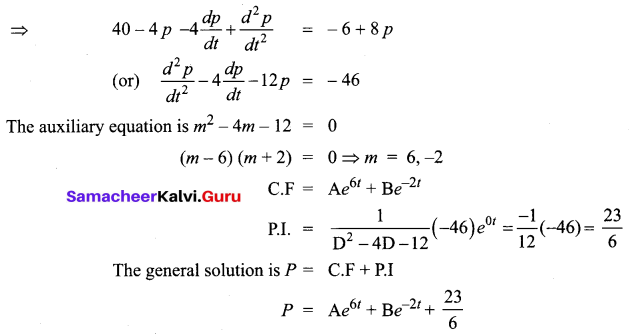# Samacheer Kalvi 12th Business Maths Solutions Chapter 4 Differential Equations Additional Problems

I. One Mark Questions

Question 1.
The differential equation of straight lines passing through the origin is ______
(a) $$\frac{x d y}{d x}=y$$
(b) $$\frac{d y}{d x}=\frac{x}{y}$$
(c) $$\frac{d y}{d x}=0$$
(d) $$\frac{x d y}{d x}=\frac{1}{y}$$
(a) $$\frac{x d y}{d x}=y$$
Hint: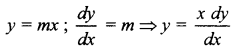Question 2.
The solution of x dy + y dx = 0 is _______
(a) x + y = c
(b) x2 + y2 = c
(c) xy = c
(d) y = cx
(c) xy = c
Hint:
x dy + y dx = 0
d(xy) = 0
xy = cQuestion 3.
The solution of x dx + y dy = 0 is _____
(a) x2 + y2 = c
(b) $$\frac{x}{y}$$ = c
(c) x2 – y2 = c
(d) xy = c
(a) x2 + y2 = c
Hint:
x dx = -y dy
$$\frac{x^{2}}{2}=\frac{-y^{2}}{2}+c_{1}$$
x2 + y2 = c

Question 4.
The solution of $$\frac{d y}{d x}$$ = ex-y is ______
(a) ey ex = c
(b) y = log cex
(c) y = log(ex + c)
(d) ex+y = c
(c) y = log(ex + c)
Hint: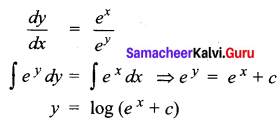Question 5.
The solution of $$\frac{d p}{d t}$$ = ke-t (k is a constant) is ________
(a) $$c-\frac{k}{e^{t}}=p$$
(b) p = ket + c
(c) t = log$$\frac{c-p}{k}$$
(d) t = logc p
(a) $$c-\frac{k}{e^{t}}=p$$
Hint: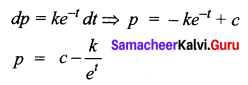Question 6.
The integrating factor of (1 + x2) $$\frac{d y}{d x}$$ + xy = (1 + x2)3 is _______
(a) $$\sqrt{1+x^{2}}$$
(b) log(1 + x2)
(c) $$e^{\tan ^{-1} x}$$
(d) $$\log ^{\left(\tan ^{-1} x\right)}$$
(a) $$\sqrt{1+x^{2}}$$
Hint: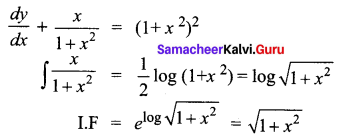Question 7.
The complementary function of the differential equation (D2 – D) y = ex is _____
(a) A + B ex
(b) (Ax + B) ex
(c) A + B e-x
(d) (A + Bx) e-x
(a) A + B ex
Hint:
m2 – m = 0
m(m – 1) = 0
CF = Ae0x + Bex = A + B exQuestion 8.
Match the following
(a) $$\frac{d^{4} y}{d x^{4}}$$ + sin y = 0 – (i) order 1, degree 1
(b) y’ + y = ex – (ii) order 3, degree 2
(c) y'” + 2y” + y’ = 0 – (iii) order 4, degree 1
(d) (y”’)2 + y’ + y5 = 0 – (iv) order 3, degree 1
(a) – (iii)
(b) – (i)
(c) – (iv)
(d) – (ii)

Question 9.
Fill in the blanks
(a) The general solution of the equation $$\frac{d y}{d x}+\frac{y}{x}=1$$ is ______
(b) Integrating factor of $$\frac{x d y}{d x}$$ – y = sin x is ______
(c) The differential equation of y = A sin x + B cos x is _______
(d) The D.E $$\frac{d y}{d x}+\frac{y}{x \log x}=\frac{1}{x}$$ is a ________ differential equation.
(a) $$y=\frac{x}{2}+\frac{c}{x}$$
(b) $$\frac{1}{x}$$
(c) $$\frac{d^{2} y}{d x^{2}}+y=0$$
(d) linearQuestion 10.
State true or false
(a) y = 3 sin x + 4 cos x is a particular solution of the differential equation $$\frac{d^{2} y}{d x^{2}}$$ + y = 0
(b) The solution of $$\frac{d y}{d x}=\frac{x+2 y}{x}$$ is x + y = kx2
(c) y = 13ex + 4e-x is a solution of $$\frac{d^{2} y}{d x^{2}}$$ – y = 0
(a) True
(b) True
(c) True

II. 2 Marks Questions

Question 1.
Form the D.E of the family of curves y = ae3x + bex where a, b are parameters
Solution:
y = ae3x + bex
$$\frac{d y}{d x}$$ = 3 ae3x + bex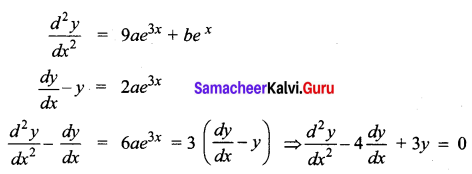Question 2.
Find the D.E of a family of curves y = a cos (mx + b), a and b are constants.
Solution: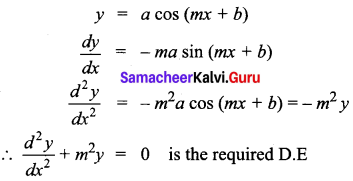Question 3.
Find the D.E by eliminating the constants a and b from y = a tan x + b sec x
Solution: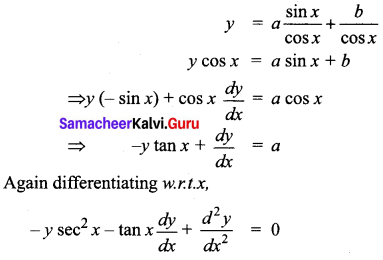Question 4.
Solve: $$\frac{d y}{d x}=e^{7 x+y}$$
Solution: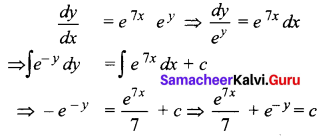Question 5.
Solve: (x2 – ay) dx = (ax – y2) dy
Solution:
Writing the equation as
x2 dx + y2 dy = a (x dy + y dx)
x2 dx + y2 dy = a d(xy)
∫x2 dx + ∫y2 dy = a ∫d(xy) + c
$$\frac{x^{3}}{3}+\frac{y^{3}}{3}$$ = axy + c
Hence the general solution is x3 + y3 = 3axy + cQuestion 6.
Solve (sin x + cos x) dy + (cos x – sin x) dx = 0
Solution:
The given equation can be written as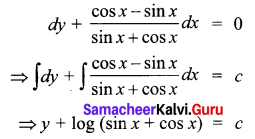III. 3 and 5 Marks Questions

Question 1.
Solve $$\frac{x d y}{d x}$$ + cos y = 0, given y = $$\frac{\pi}{4}$$ when x = √2
Solution: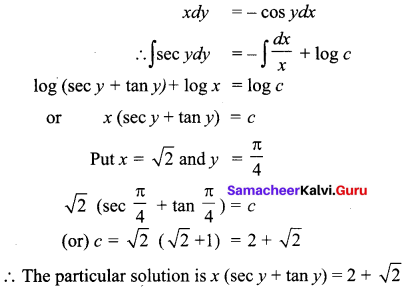Question 2.
The slope of a curve at any point is the reciprocal of twice the ordinate of the point. The curve also passes through the point (4, 3). Find the equation of the curve.
Solution:
Slope at any point (x, y) is the slope of the tangent at (x, y)
$$\frac{d y}{d x}=\frac{1}{2 y}$$
⇒ 2y dy = dx
⇒ ∫2y dy = ∫dx + c
⇒ y2 = x + c
Since the curve passes through (4, 3)
we have 9 = 4 + c ⇒ c = 5
Equation of the curve is y2 = x + 5Question 3.
The net profit P and quantity x satisfy the differential equation $$\frac{d p}{d x}=\frac{2 p^{3}-x^{3}}{3 x p^{2}}$$. Find the relationship between the net profit and demand given that p = 20 when x = 10
Solution: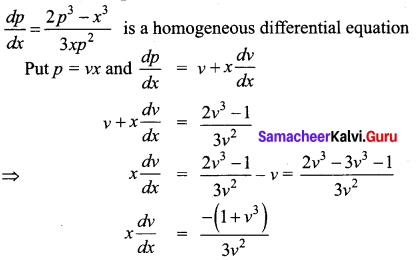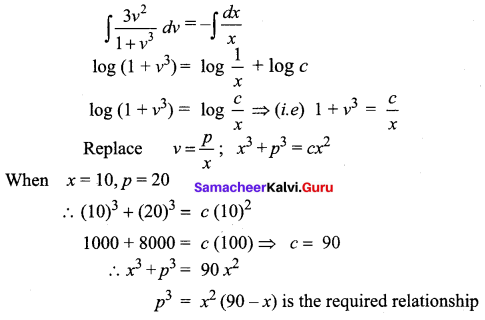Question 4.
Solve: $$\frac{d y}{d x}$$ + ay = ex (a ≠ -1)
Solution:
The given equation is of the form $$\frac{d y}{d x}$$ + Py = Q
Here P = a, Q = ex
The general solution is y (I.F) = ∫Q (I.F) dx + c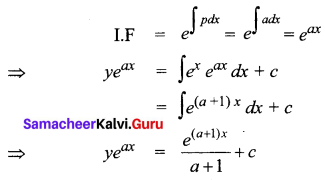Question 5.
Solve: $$\frac{d y}{d x}$$ + y cos x = $$\frac{1}{2}$$ sin 2x
Solution:
Here P = cos x, Q = $$\frac{1}{2}$$ sin 2x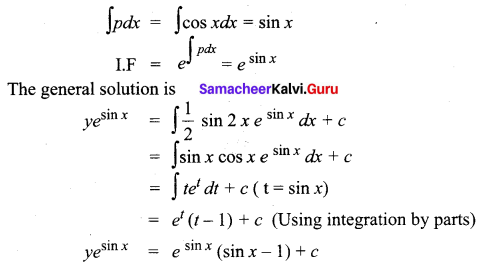Question 6.
Solve (D2 – 6D + 25) y = 0
Solution:
The auxiliary equations is m2 – 6m + 25 = 0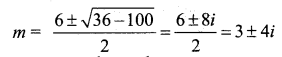The Roots are complex and of the form,
α ± β with α = 3 and β = 4
The complementary function = e3x (A cos 4x + B sin 4x)
The general solution is y = e3x (A cos 4x + B sin 4x)Question 7.
Solve (D2 + 10D + 25) y = $$\frac{5}{2}$$ + e-5x
Solution:
The auxiliary equations is m2 + 10m + 25 = 0
(m + 5)2 = 0
m = -5, -5
The complementary function = (Ax + B) e-5x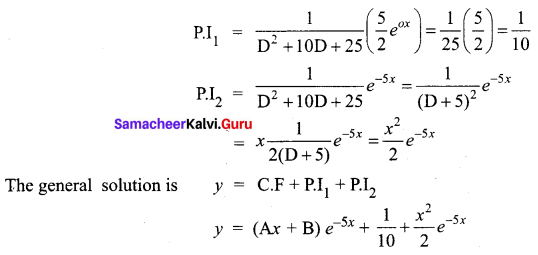Question 8.
Suppose that the quantity demanded Qd = 40 – 4p – 4$$\frac{d p}{d t}+\frac{d^{2} p}{d t^{2}}$$ and quantity supplied Qs = -6 + 8p where p is the price. Find the equilibrium price for market clearance.
Solution:
For market clearance, the required condition is Qd = Qs